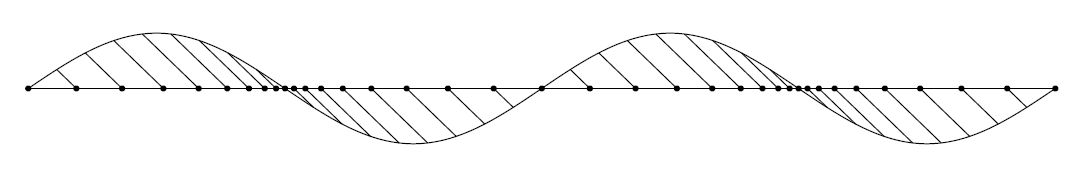## How to construct longitudinal from transversal waves and vice versa?The above construction of a longitudinal wave out of a transversal wave has been encountered somewhere in an old physics textbook. There are several drawbacks with this construction.
• The maximum densities of the longitudinal wave are not where you would expect them, namely at the extrema of the transversal wave.
• More seriously. There is a restriction on the maximum amplitude $A$ of the transversal wave, namely $A < \lambda/(2\pi)$, where $\lambda$ is the wavelength.
Proof of the latter. It's obvious from the picture that the slope of the tangent line at any point of the transversal wave must be greater than minus one. This requirement is most critical e.g. at half the wavelength. Hence: $$T(x) = A.\sin\left(\frac{2\pi}{\lambda} x \right) \quad \Longrightarrow \quad T'(x) = A.\frac{2\pi}{\lambda} \cos\left(\frac{2\pi}{\lambda} x \right) \quad \Longrightarrow \quad T'\left(\frac{\lambda}{2}\right) = A.\frac{2\pi}{\lambda} \cos(\pi) = - A.\frac{2\pi}{\lambda} > -1 \quad \Longrightarrow \quad A < \frac{\lambda}{2\pi}$$ Question. Are there better ways to construct longitudinal waves from transversal waves? And is also it possible to do it the other way around: construct a (continuous) transversal wave out of a (discrete) longitudinal wave? Here it is noted that the longitudinal wave, in mathematical terms, is actually a monotone sequence of points $x_k$ instead of a function.
After some thought, it seems that the comment by dfeuer is quite to the point: "what properties you want this construction to have?" A good question is half the answer. So here comes my wish list:
1. the sequence of points $x_k$ must represent a longitudinal wave
2. the sequence $x_k$ must must be monotonically increasing
3. the construction must be independent of the discretization $\Delta$
A (sinusoidal) longitudinal wave is given by the formula: $$x_k = k\Delta + A.\sin\left(\frac{2\pi}{\lambda}k\Delta\right) \quad ; \quad k \in \mathbb{N}$$ Which is generated exactly by the construction as presented in the question.
Monotonically increasing means that $x_{k+1} > x_k$ , giving: $$(k+1)\Delta + A.\sin\left(\frac{2\pi}{\lambda}(k+1)\Delta\right) > k\Delta + A.\sin\left(\frac{2\pi}{\lambda}k\Delta\right)$$ With help of the formula $\;\sin(p)-\sin(q)=2.\cos\frac{1}{2}(p+q).\sin\frac{1}{2}(p-q)\;$ we find: $$\Delta + 2.A.\cos\left(\frac{2\pi}{\lambda}k\Delta + \frac{\pi}{\lambda}\Delta\right) .\sin\left(\frac{\pi}{\lambda}\Delta\right) > 0$$ This must be independent of $k$ in the first place. Worst case scenario: $\;\cos\left(\frac{2\pi}{\lambda}k\Delta+\frac{\pi}{\lambda}\Delta\right) = -1\;$. Giving, while anticipating a bit on the next requirement: $$\Delta - 2.A.\left[\frac{\sin\left(\frac{\pi}{\lambda}\Delta\right)}{\frac{\pi}{\lambda}\Delta}\right] \frac{\pi}{\lambda}\Delta > 0$$ Let's assume that the discretization $\Delta$ is reasonable with respect to the wavelength $\lambda$, say $\Delta < \lambda/4$. This is no serious restriction. Then the worst case scenario for $\Delta$ is that it becomes infinitesimally small. Because we know that $$\frac{\sin\left(\frac{\pi}{\lambda}\Delta\right)}{\frac{\pi}{\lambda}\Delta} < 1 \qquad \mbox{and} \qquad \lim_{\Delta \to 0} \frac{\sin\left(\frac{\pi}{\lambda}\Delta\right)}{\frac{\pi}{\lambda}\Delta} = 1$$ Consequently, if all of our wishes are fulfilled, there is a restriction on the amplitude of longitudinal waves, exactly the same one as has already been derived in the question for the corresponding transversal wave. And it is independent of the discretization $\Delta$: $$\Delta - 2.A.\frac{\pi}{\lambda}\Delta > 0 \qquad \Longrightarrow \qquad A < \frac{\lambda}{2\pi}$$ Most astonishing is that I have never encountered this restriction anywhere in literature.